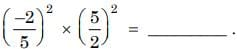# Test: Exponents and Powers- 1

## 10 Questions MCQ Test Mental Mathematics | Test: Exponents and Powers- 1

Description
Attempt Test: Exponents and Powers- 1 | 10 questions in 20 minutes | Mock test for Class 7 preparation | Free important questions MCQ to study Mental Mathematics for Class 7 Exam | Download free PDF with solutions
QUESTION: 1

Solution:
QUESTION: 2

Solution:
QUESTION: 3

### FindSolution:
QUESTION: 4

Find the value of (32)2.

Solution:
QUESTION: 5

Calculate 25 + 52

Solution:
QUESTION: 6

Find the value of 32 × 23

Solution:
QUESTION: 7

If (x5 × x–2)2 = 64, what is x?

Solution:
QUESTION: 8

13 + 1–3 = ?

Solution:
QUESTION: 9

Find the value (211 + 32 – 51)0.

Solution:
QUESTION: 10

Find the value of a2b3 when a = 2 and b = 3.

Solution:Use Code STAYHOME200 and get INR 200 additional OFF Use Coupon Code